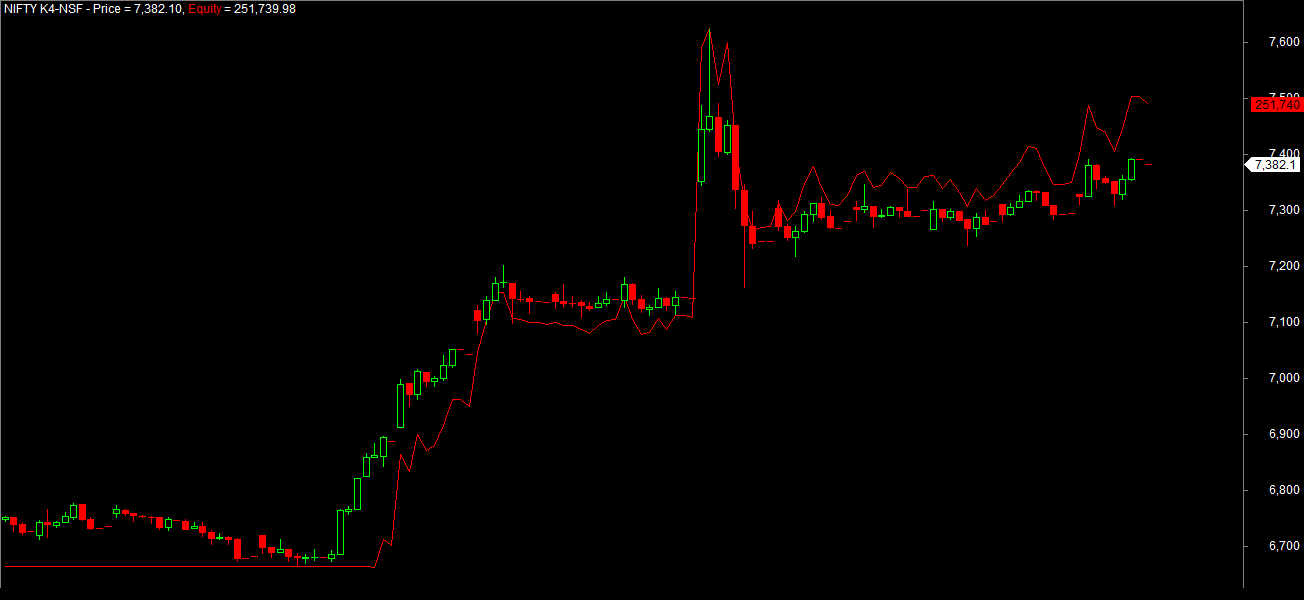# How to Plot the Equity Curve without Backtesting?

Equity Curve is the graphical representation of how your money grows when you follow a particular trading system over a period of time. It shows whether your trading strategy is making money or loosing over a period of time.

In Amibroker the general way of computing the Equity curve is to backtest the given trading system and the report computes the equity curve, drawdown…etc results for you. And to compute the equity curve you have to push the backtest button every time which makes you little uncomfortable if you want to measure the Equity curve in realtime.

Here is a simple example which solves the problem and makes to measure the Equity curve to be computed in realtime. You can use this as a template in your trading strategies to calculate and plot the equity curve in realtime.

Set the Initial Parameters

Let us assume that we have a Initial capital of Rs2,00,000 and we want to test our trading system in Nifty futures(10% Margin deposit Required) and Rs100 for per leg transaction for two lots of Nifty Futures(i.e Rs200 for both buy and Sell. Then the Intial Parameters can be set using the AFL code as shown below

//Initial Parameters

SetOption( “InitialEquity”, 200000);
SetOption(“FuturesMode” ,True);
SetOption(“MinShares”,1);
SetOption(“CommissionMode”,2);
SetOption(“CommissionAmount”,100);
SetOption(“AccountMargin”,10);
SetOption(“RefreshWhenCompleted”,True);

//Position Sizing

SetPositionSize(100,spsShares);

Once the initial parameters are set then you need to concentrate on your buy and sell logic. Here in this case i had taken a simple EMA crossover 20 and 50 in this example for easier understanding and the logic is code as afl as shown below.

Rules are very Simple Buy when 20 EMA crosses 50EMA on the upper side(Positive Crossover) and sell when 50EMA crosses 20 EMA on the downside(negative crossover).

//Parameters

MALength1 = Optimize(“MALength1”,20,1,5,1);
MALength2 = Optimize(“MALength2”,50,1,5,1);

Buy = Cross(ema( C, MALength1 ),ema( C, MALength2 ));
Sell =Cross( ema( C, MALength2 ), ema( C, MALength1 )) ;

Sell = ExRem( Sell, Buy );

Short = Sell;

Compute Equity Curve

In Amibroker the equity function returns single-security Equity line based on buy/sell/short/cover rules, buy/sell/short/coverprice arrays, all apply stops, and all other backtester settings.

For Example e=equity(0,1); retrieves the equity curve for based on buy/sell/short/cover rules with the given initial parameters. however if you want to retrieve the equity curve only for the selected from and to dates then checkout the following example which computes the equity curve between 1st May 2014 to 31st May 2014 and it computes in realtime.

start = ParamDate( “Start Date”, “2014-05-01” );
end=ParamDate( “End Date”, “2014-05-31” );
e=Equity(1,3,start,end); //Calculates for the Date Specific Quotes

For more detailed information refer the Equity Function in Amibroker.Image showing the Nifty May futures hourly charts along with the Equity curve since 1st May 2014.

[note: Code provided here is for illustration purpose only]## How to Perform Machine Learning Using Amibroker and Python

Amibroker is a powerful technical analysis and trading system development platform, used extensively by traders and analysts for developing and deploying trading strategies. Python,...

## Preparing the Features Dataset using Amibroker Exploration – Machine…

There are various methods to prepare the feature dataset, which is a crucial input for a machine learning prediction model. One approach is to...

## Dynamic Color Coding in Amibroker Profit Tables: A Visually…

Profit tables are a valuable tool for traders and investors to analyze the trading strategy performance of their investments over time. However, the traditional...

## 2 Replies to “How to Plot the Equity Curve without Backtesting?”

1.k.kathiresan says:

1.Rajandran R says: